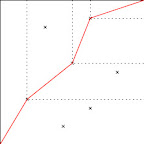# Maximal directed crossing of a box using uniform random variables

Take a 1 by 1 box $D \subset \mathbb{R}^2$ and let $U_1,\dots,U_n$ be i.i.d. uniforms in $D$.

Suppose at the start all of $\mathcal{V}_0=\{U_1,\dots,U_n\}$ are viable. At each step pick one of the viable uniform random variables $U=(U^1,U^2)$ according to some strategy. Say this point is eaten. The viable points are now $\mathcal{V}_1:=\{V=(V^1,V^2) \in \mathcal{V}: V^1\geq U^1 \text{ and } V^2 \geq U^2\}$. Carry on in this manner until there no more viable points.

Let $M(n)$ denote the maximum number of eaten points over all strategies. This is the maximal number of points visited on a directed crossing from one corner of the box to the opposite corner.

I am interested in the behavior of $\mathbb{E}[M(n)]$ asymptotically in $n$.

I have a picture of this below. Here, there are many strategies that maximise the number of eaten points, which is 3, one of which is drawn in red.I expect that $\mathbb{E}[M(n)] \approx C\log n$ as it seems that for an optimal strategy the proportion of points $\frac{|\mathcal{V}_{i+1}|}{|\mathcal{V}_i|}$ lost by eating a point remains roughly the same. I am having trouble showing this rigorously.

The problem that you are asking is actually very well known in probability theory. First of all, notice that $M(n)$ has the same distribution as the longest increasing subsequence in a random permutation of $S_n$. To see this, note that if the $n$ points are ordered according to their $x$-coordinate values, then the corresponding orders of the $y$-coordinates gives a random permutation and all permutations are equally likely.
A lot of very interesting research has been done on the distribution of $M(n)$ for large $n$. In particular, to answer your question it is known that $$\lim_{n\rightarrow \infty} \frac{E[M(n)]}{\sqrt{n}} = 2.$$ Much more, however, is known about the distribution of $E[M(n)]$. For instance, the paper "On the distribution of the length of the longest increasing subsequence of random permutations" by Baik, Deift, and Johansson (1999) is a very important result, showing that the distribution of $\frac{M(n) - 2\sqrt{n}}{n^{1/6}}$ converges in distribution to the Tracy-Widom distribution from random matrix theory.